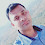# Data Science Introduction

2

Data science is a way to try and discover hidden patterns in raw data. To achieve this goal, it makes use of several algorithms, machine learning(ML) principles, and scientific methods. The insights it retrieves from data lie in forms structured and unstructured. So in a way, this is like data mining. Data science encompasses all- data analysis, statistics, and machine learning. With more practices being labelled into data science.

Text Analysis
Statistical Analysis
Diagnostic Analysis
Predictive Analysis
Prescriptive Analysis

TEXT ANALYSIS:-
Text Analysis is also referred to as Data Mining. It is a method to discover a pattern in large data sets using databases or data mining tools. It used to transform raw data into business information. Business Intelligence tools are present in the market which is used to take strategic business decisions. Overall it offers a way to extract and examine data and deriving patterns and finally interpretation of the data.
Statistical Analysis
It is used to analyze statistical data. Statistical Analysis includes the collection, analysis, interpretation, presentation, and modeling of data. It analyses a set of data or a sample of data. There are two categories of this type of Analysis - Descriptive Analysis and Inferential Analysis.
Descriptive Analysis
analyses complete data or a sample of summarized numerical data. It shows mean and deviation for continuous data whereas percentage and frequency for categorical data
Inferential Analysis
analyses sample from complete data. In this type of Analysis, you can find different conclusions from the same data by selecting different samples
Diagnostic Analysis
Diagnostic Analysis shows "Why did it happen?" by finding the cause from the insight found in Statistical Analysis. This Analysis is useful to identify behavior patterns of data. If a new problem arrives in your business process, then you can look into this Analysis to find similar patterns of that problem. And it may have chances to use similar prescriptions for the new problems.
Predictive Analysis
Predictive Analysis shows "what is likely to happen" by using previous data. The simplest example is like if last year I bought two dresses based on my savings and if this year my salary is increasing double then I can buy four dresses. But of course it's not easy like this because you have to think about other circumstances like chances of prices of clothes is increased this year or maybe instead of dresses you want to buy a new bike, or you need to buy a house!
So here, this Analysis makes predictions about future outcomes based on current or past data. Forecasting is just an estimate. Its accuracy is based on how much detailed information you have and how much you dig in it.
Prescriptive Analysis:-
Prescriptive Analysis combines the insight from all previous Analyses to determine which action to take in a current problem or decision. Most data-driven companies are utilizing Prescriptive Analysis because predictive and descriptive Analysis are not enough to improve data performance. Based on current situations and problems, they analyze the data and make decisions.

1.# DATA SCIENCE ( 7 To 8 PM BATCH )
# Program to Calculate the age based on the Date of Birth .

import datetime
#from datetime import date

ye = int(input("Please Enter Birth Year :-"))
mo = int(input("Please Enter Birth Month :-"))
da = int(input("Please Enter Birth Day :-"))

dob = datetime.date(ye, mo, da)

cd = datetime.date.today()

time_difference = end_date - birth_date

age = time_difference.days

y = 0

print("Total Days:-",age)

a = age//365
b = age%365
c = b//30
if cd.day>=dob.day:
y = cd.day-dob.day
else:
y = (cd.day+30)-dob.day

print("Age in Year ,Month and Day respectively :-",a,c,y)

2.import pytesseract
from PIL import Image
import os
from gtts import gTTS
import pyttsx3

img = Image.open('p://image//si.png')
print(img)
pytesseract.pytesseract.tesseract_cmd ='C://Users//DELL//AppData//Local//Tesseract-OCR//tesseract.exe'
result = pytesseract.image_to_string(img)
with open('abc.txt',mode ='w+') as file:
file.write(result)
print(result)
engine = pyttsx3.init()
engine.say(result)
engine.runAndWait()
rate = engine.getProperty("rate")
print(rate)

engine.setProperty("rate", 300)
engine.say(result)
engine.runAndWait()
engine.setProperty("rate", 100)
engine.say(result)
engine.runAndWait()